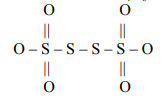One of the products of the following reaction $KCNO+{\left(NH\right)}_{4}S{O}_{4}$ is :

(A) $N{H}_{4}N{O}_{2}$                              (B) $N{H}_{4}N{O}_{3}$

(C) $N{H}_{2}-CO-N{H}_{2}$                  (D) ${N}_{2}$ and $N{O}_{2}$ both

Concept Questions :-

group 15 ,preparation and properties
High Yielding Test Series + Question Bank - NEET 2020

Difficulty Level:

Which of the following orders is correct?

(1) $Sb{H}_{3}>N{H}_{3}>As{H}_{3}>P{H}_{3}$ – Boiling Point

(2) $N{H}_{3}>P{H}_{3}>As{H}_{3}>Sb{H}_{3}$  – Thermal Stability

(3) $N{H}_{3}>P{H}_{3}>As{H}_{3}>Sb{H}_{3}$  – Basic Character

(4) $N{H}_{3}>P{H}_{3}>As{H}_{3}>Sb{H}_{3}$  – Bond Angle

(A) (1), (2) and (3) only                             (B) (2), (3) and (4) only

(C) (1), (3) and (4) only                             (D) (1), (2), (3) and (4).

Concept Questions :-

group 15 ,preparation and properties
High Yielding Test Series + Question Bank - NEET 2020

Difficulty Level:

Which of the following is correct?

(A) ${S}_{3}{O}_{9}$  – contains no S - S linkage.

(B) ${S}_{2}{O}_{6}^{2-}$   – contains – O - O linkage.

(C) ${\left(HP{O}_{3}\right)}_{3}$  – contains P – P linkage.

(D) ${S}_{2}{O}_{8}^{2-}$ - contains S – S linkage.

Concept Questions :-

group 16,preparation and properties
High Yielding Test Series + Question Bank - NEET 2020

Difficulty Level:

The oxidation states of S atom in ${S}_{4}{O}_{6}^{2-}$  from left to right respectively are:(A) + 6, 0, 0, +6                          (B) +5, +1, +1, +5

(C) +5, 0, 0, +5                           (D) +3, +1, +1, +3

Concept Questions :-

group 16,preparation and properties
High Yielding Test Series + Question Bank - NEET 2020

Difficulty Level:

One gas bleaches the coloured matter by reduction temporary while the other one by oxidation
permanently. The gases are respectively:

(A)                               (B)

(C)                              (D)

Concept Questions :-

group 16,preparation and properties
High Yielding Test Series + Question Bank - NEET 2020

Difficulty Level:

${H}_{2}S{O}_{4}$  cannot be used to prepare HI from KI as:

(A) Its reaction with KI is very slow.                   (B) It oxidizes HI to iodine.
(C) It disproportionates HI.                                (D) It reduces HI.

Concept Questions :-

group 17 ,preparation and properties
High Yielding Test Series + Question Bank - NEET 2020

Difficulty Level:

Which of the following statements is false?

(A)   all are tribasic and reducing in nature.

(B) Amongst anions

(C)  Amongst anions   is basic and reducing in nature.

(D) Number of lone pair(s) of electrons on ${X}_{3}$ atoms in  are 3, 2 and 1 respectively.

Concept Questions :-

Oxyacids of Phosphorus/Nitrogen
High Yielding Test Series + Question Bank - NEET 2020

Difficulty Level:

Which one of the following statements regarding helium is incorrect?
(A) It is used to produce and sustain powerful superconducting magnets.
(B) It is used as a cryogenic agent for carrying out experiments at low temperatures.
(C) It is used in filling balloons for meterological observations because it is lighter and non-inflammable.
(D) It is used as dilute for oxygen in modern diving apparatus because of its high solubility in blood.

Concept Questions :-

group 18,perparation and properties
High Yielding Test Series + Question Bank - NEET 2020

Difficulty Level:

Assertion : $Pb{I}_{4}$  is not known probably because of the oxidising power of Pb(IV) and reducing
power of ${I}^{-}$.

Reason :  Iodide stabilizes higher oxidation state.

1. If both the assertion and the reason are true and the reason is a correct explanation of the assertion
2. If both the assertion and reason are true but the reason is not a correct explanation of the assertion
3. If the assertion is true but the reason is false
4. If both the assertion and reason are false

Concept Questions :-

group 15 ,preparation and properties
High Yielding Test Series + Question Bank - NEET 2020

Difficulty Level:

Assertion : ${P}_{4}{O}_{10}$ is not used to dry $N{H}_{3}$.

Reason : ${P}_{4}{O}_{10}$ is acidic and $N{H}_{3}$ is basic.

1. If both the assertion and the reason are true and the reason is a correct explanation of the assertion
2. If both the assertion and reason are true but the reason is not a correct explanation of the assertion
3. If the assertion is true but the reason is false
4. If both the assertion and reason are false

Concept Questions :-

group 15 ,preparation and properties